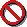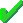# The "Let's Make a Deal" (Monty Hall) Problem

Turning word problems into probability problems can be subtle, and intuition about probability can be misleading. This chapter looks carefully at a problem that has confused both the general public and professional mathematicians and statisticians: the Let's Make a Deal or Monty Hall problem. At issue is whether the conditional probability of two events is equal.

## Background

"Let's make a Deal" is a game show on television. In one of the games, a contestant tries to guess which of three doors hides a prize. After the contestant chooses, the host, Monty Hall, reveals to the contestant that the prize is not behind one of the doors the contestant did not pick; Monty then offers the contestant the opportunity to change her guess to the remaining door.

Whether it is better strategy at that point to switch one's guess is a question that has generated some publicity: Marilyn vos Savant, "the world's smartest person," has a syndicated column called "Ask Marilyn," in which she said that switching is the better strategy. A number of professors wrote to her with proofs that switching does not help.

is an implementation of the game. The question marks represent the three doors. Click one of the question marks; what is behind another door will be revealed:means the prize was not behind that door.means the prize was behind the door.

Either click the same door again (stick with your original guess), or switch your guess by clicking the other closed door. What was behind the two unopened doors then will be revealed. To play again, click any door, or click the New Game button.

Be assured that the game is programmed so that the prize is hidden at random before you pick a door, with chance 1/3 of being behind each of the doors. The prize stays put until you start a new game; it is then placed behind one of the doors at random again.

We shall take a closer look at the question of the better strategy (stick with the original guess, or switch to the other unopened door), from several points of view.

## Assumptions and Arguments

We shall begin by making the rules of the game precise, so that we can compare the arguments carefully. Then we shall examine two arguments against switching—one naive and one based on conditional probability—and two arguments for switching—one heuristic and one based on conditional probability.

### Assumptions and Rules of the Game

We shall assume that the game is played as follows:

1. The prize is hidden randomly, with chance 1/3 of being behind each of the three doors.
2. The contestant picks a door at random, with chance 1/3 of picking each door.
3. The contestant's choice is independent of the door the prize is really behind.
4. After the contestant guesses, the host always reveals that the prize is not behind one of the two doors the contestant did not pick. If the contestant happens to pick the correct door the first time, the host chooses which of the other two doors to reveal randomly, with chance 50% each.

The game in this chapter satisfies assumptions (1) and (4), and, neglecting paranormal abilities on your part, also satisfies (3). I have no way to know whether your way of picking a door satisfies (2) or not, but in fact, the assumption is not needed—we could eliminate assumptions 2 and 3 by conditioning on the door the contestant picks, and the analysis would be essentially the same. With these assumptions, we are ready for the arguments.

### Argument 1 (don't switch—naive)

Argument 1 says it cannot help to switch: After the host reveals what is behind one door, there are two doors left, and the prize is equally likely to be behind either one. Therefore, there is no advantage to switching

### Argument 2 (don't switch—conditional probability)

Argument 2 is a more sophisticated version of Argument 1, using conditional probability. It also concludes there's no advantage to switching. I think this is the argument that the professors had in mind.

Label the three doors a, b, and c. Let A be the event that the prize is behind door a, B be the event that the prize is behind b, etc. To keep things definite, we assume the contestant picks a; the host then reveals either Bc or Cc, that is, that the prize is not behind b or that the prize is not behind c. For definiteness, suppose the host reveals Bc.

According to Argument 2, the contestant should switch if the conditional probability of C given Bc is larger than the conditional probability of A given Bc, because then the chance of winning, having learned that the prize is not behind door b, is higher if the contestant switches. Here is the algebra:

P(C | Bc) = P(CBc)/P(Bc)

= P(C)/P(Bc)

= (1/3)/(2/3)

= 1/2.

The second line uses the fact that CBc = C: The only way that the prize can be behind door c is if it is not behind door b, so C is a subset of Bc, and hence CBc = C. By similar reasoning,

P(A | Bc) = P(ABc)/P(Bc)

= P(A)/P(Bc)

= (1/3)/(2/3)

= 1/2.

Thus there is no advantage to switching.

### Argument 3 (switch—heuristic)

Marilyn's argument went something like this:

Suppose you play over and over. In the long run, 1/3 of the time, your first guess will be right. So, if you never switch, you will win 1/3 of the time. But when your first guess is wrong (which happens 2/3 of the time in the long run), you will win if you switch. So, if you switch every time, you will win 2/3 of the time in the long run. Thus you should switch.

This argument essentially applies the Frequency Theory of Probability to determine the probability of winning by a particular strategy: If, in the long run, a contestant would win 2/3 of the time by switching, the probability of winning by switching is 2/3. Marilyn's argument seems pretty persuasive, but what about the more mathematical argument using conditional probability? Why do they disagree? Doesn't the mathematical argument have to be right?

Actually, Argument 2 calculates a conditional probability that has little to do with how the game is played. In the notation we used in Argument 2, the event that we condition on should not be Bc. The contestant does not learn "out of the blue" that the prize is not behind door b. Rather, the host's choice to open door b depends on which door the contestant picked, and on which door the prize is really behind. This leads us to the fourth argument.

### Argument 4 (switch—conditional probability)

According to the fourth argument, the conditional probability we seek is actually

P(C | {pick a} and {host reveals Bc}).

To calculate this conditional probability, we need to use the rest of the assumptions; only the first was needed for the other arguments. Under the assumptions, {C and {pick a}} implies {host reveals Bc}, because b is the only door the host can open without revealing the prize. Thus the event {C and {pick a} } is a subset of the event {host reveals Bc}. The chance of the event {C and {pick a} } is

P(C and {pick a} ) = 1/3 × 1/3 = 1/9

using our assumption that the random hiding of the prize is independent of the contestant's choice of a door.

The chance of the event

{ {pick a} and {host reveals Bc} }

can be found as follows. The event can occur two ways: Either the prize really is behind door a or really is behind door c; these possibilities are mutually exclusive, so they partition the event { {pick a} and {host reveals Bc} }. Hence,

P({pick a} and {host reveals Bc}) =

P(A and {pick a} and {host reveals Bc}) + P(C and {pick a} and {host reveals Bc}).

The latter summand is 1/9, as we just saw. We can find the first summand using the Multiplication Rule for conditional probabilities:

P(A and {pick a} and {host reveals Bc}) =

P({host reveals Bc} | A and {pick a}) × P(A and {pick a})

= 1/2 × 1/9 = 1/18

using the assumption that the host decides randomly which door to reveal if the contestant picked the right door the first time, and the assumption that the contestant's choice of door and the hiding of the prize behind a door are independent. We thus find

P(C | {pick a} and {host reveals Bc}) =

P(C and {pick a} and {host reveals Bc})/P({pick a} and {host reveals Bc})

= (1/9)/(1/9 + 1/18)

= 2/3.

This agrees with Marilyn's conclusion: switching is better.

## Conclusions

Which of these arguments do you find most satisfactory? Most intuitive?

• Argument 1 jumps to the fallacious conclusion that if there are two outcomes, they must be equally likely.
• Argument 2 misuses conditional probability, by not recognizing that the host's choice of door to open depends on which door the contestant picks.
• Argument 3 is simple, and depends only on the assumption that the prize initially is equally likely to be behind any of the three doors.
• Argument 4 is technical, and requires additional assumptions about independence, how the host decides which door to show when the contestant picked the right one in the first place, etc.

In we will construct an empirical approach to testing which conclusion is correct.

The following exercises check your ability to extend the reasoning we used in this chapter to more complicated situations.

## Summary

Intuition about probability can be misleading; so can careless probability calculations. Conditional probability can be subtle, especially when turning a verbal story into a mathematical problem: Be sure to condition on the right event. Thinking about probabilities using frequentist ideas—considering what would happen in repeated trials—sometimes can guide your intuition about probability.

## Key Terms

• conditional probability
• event
• Frequency Theory of Probability
• independent
• Multiplication Rule
• mutually exclusive
• probability